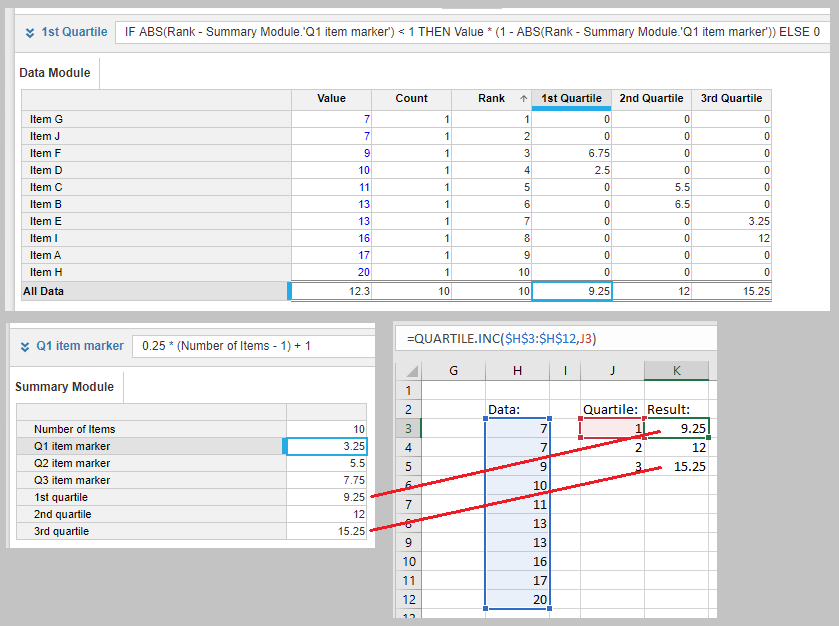# Creating QUARTILE function within AnaplanThis is a method of building the Excel “Quartile” function within Anaplan – it requires 11 Line items, requires that the Data dimension has a ‘top level’ value, and uses the RANK function which has an upper limit of 50 million cells. To start, create a module (or use an existing calc module) dimensioned by your base data and whatever dimensions are necessary. In my example, I used just a single "Data List" dimension.

In that module, add the following formulas:

Data Module

Count = 1

Rank = RANK({Value} <wherever that is in the model>, ASCENDING, SEQUENTIAL)

The count summary method must be set to Sum, the Rank summary method can be set to None.

Then create a summary module, without the base data dimension but all other dimensions (if any) the same.

If you are replicating the QUARTILE.INC then add the following formulas:

Summary Module

Number of Items = Data Module.Count

Q1 item marker = 0.25 * (Number of Items - 1) + 1

Q2 item marker = 0.5 * (Number of Items - 1) + 1

Q3 item marker = 0.75 * (Number of Items - 1) + 1

If you are replicating the QUARTILE.EXC then the section after the percentage value should be changed to * (Number of Items + 1) for all three item marker formulas – For example:
Q1 item marker = 0.25 * (Number of Items + 1)

This now tells us which ranked item should selected for the Median (Q2), First Quartile (Q1), and Top Quartile (Q3) values.

Then go back to the Data Modules and we will build three line items that pull out the correct items, or interpolate between them if necessary:

Data Module

1st Quartile = IF ABS(Rank - Summary Module.'Q1 item marker') < 1 THEN {Value} * (1 - ABS(Rank - Summary Module.'Q1 item marker')) ELSE 0

2nd Quartile = IF ABS(Rank - Summary Module.'Q2 item marker') < 1 THEN {Value} * (1 - ABS(Rank - Summary Module.'Q2 item marker')) ELSE 0

3rd Quartile = IF ABS(Rank - Summary Module.'Q3 item marker') < 1 THEN {Value} * (1 - ABS(Rank - Summary Module.'Q3 item marker')) ELSE 0

These must all have the summary method of Sum. The IF clause in this formula will only pull through values whose rank matches the appropriate median/quartile value, and the THEN clause will take that only the specific value if it lands exactly, or interpolate in situations where the median lies between two values, and the two partial values will be automatically added up by the summary method of “Sum”.

Then finally, in the Summary Module, we add 3 more line items to pull the correct summarized value. Since the Data dimension is not present in the Summary Module, the default behavior is to take the top level summarized value:

Summary Module

1st quartile = Data Module.'1st Quartile'

2nd quartile = Data Module.'2nd Quartile'

3rd quartile = Data Module.'3rd Quartile'

From there, those summarized line items can be used however is needed downstream.

One thing to note, if only the Top Quartile value is needed, then the Q1 and Q2 line items in both the Data and Summary module can be removed.

Screenshots of my Data and Summary modules with a very simple data set, recreating the QUARTILE.INC function, and comparing it to the Excel result:Tagged: